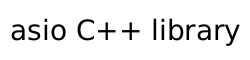Resumable C++ 20 Coroutines

The experimental::coro class provides support for a universal C++20 coroutine. The can be used as tasks, generators and transfomers, depending on their signature.

{
while (stream.is_open())
{
std::array<char, 4096> buf;

asio::buffer(buf), experimental::use_coro);

continue;

co_yield std::string_view { buf.data(), read };
}
}

coro<void, std::size_t> line_logger(tcp::socket stream)
{
while (auto l = co_await reader)
{
std::cout << "Read: '" << *l << "'" << std::endl;
}
}

{
co_spawn(line_logger(std::move(sock),
[](std::exception_ptr, std::size_t lines)
{
std::clog << "Read " << lines << " lines" << std::endl;
}));
}

A coro is highly configurable, so that it can cover a set of different use cases.

template<
typename Yield,
typename Return = void,
typename Executor = any_io_executor>
struct coro;

Yield

The Yield parameter designates how a co_yield statement behaves. It can either be a type, like int or a signature with zero or one types:

coro<void> // A coroutine with no yield
coro<int> // A coroutine that can yield int

coro<void()> // A coroutine with no yield
coro<int()> // A coroutine that can yield int

coro<int(double)> // A coroutine that can yield int and receive double

Receiving a value means that the co_yield statement returns a value.

coro<int(int)> my_sum(any_io_executor)
{
int value = 0;
while (true)
value += co_yield value; //sum up all values
}

Putting values into a coroutine can be done it two ways: either by direct resumption (from another coro) or through async_resume. The first value gets ignored because the coroutines are lazy.

coro<void> c(any_io_executor exec)
{
auto sum = my_sum(exec);
assert(0  == co_await sum(-1));
assert(0  == co_await sum(10));
assert(10 == co_await sum(15));
assert(25 == co_await sum(0));
}

awaitable<void> a()
{
auto sum = my_sum(co_await this_coro::executor);
assert(0  == co_await sum.async_resume(-1, use_awaitable));
assert(0  == co_await sum.async_resume(10, use_awaitable));
assert(10 == co_await sum.async_resume(15, use_awaitable));
assert(25 == co_await sum.async_resume(0, use_awaitable));
}

noexcept

A coro may be noexcept:

coro<void() noexcept> c;
coro<int() noexcept> c;
coro<int(double) noexcept> c;

This will change its @c async_resume signature, from void(std::exception_ptr) to void() or void(std::exception_ptr, T) to void(T). A noexcept coro that ends with an exception will cause std::terminate to be called.

Furthermore, calls of async_resume and co_await of an expired noexcept coro will cause undefined behaviour.

Return

A coro can also define a type that can be used with co_return:

coro<void() noexcept, int> c(any_io_executor)
{
co_return 42;
}

A coro can have both a Yield and Return that are non void at the same time.

Result

The result type of a coroutine is dermined by both Yield and Return. Note that in the follwing table only the yield output value is considered, i.e. T(U) means T.

Table 2. :result_type Result type deduction

Yield

Return

noexcept

result_type

completion_signature

T

U

false

variant<T, U>

void(std::exception_ptr, variant<T, U>)

T

U

true

variant<T, U>

void(variant<T, U>)

T

void

false

optional<T>

void(std::exception_ptr, optional<T>)

T

void

true

optional<T>

void(optional<T>)

void

void

false

optional<T>

void(std::exception_ptr)

void

void

true

optional<T>

void()

void

T

false

optional<T>

void(std::exception_ptr, T)

void

T

true

optional<T>

void(T)

Executor

Every coroutine needs to have its own executor. Since the coroutine gets called multiple times, it cannot take the executor from the caller like an awaitable. Therefore a coro requires to get an executor or an execution_context passed in as the first parameter.

coro<int> with_executor(any_io_executor);
coro<int> with_context(io_context &);

It is to note, that an execution_context is defined as loosely as possible. An execution context is any object that has a get_executor() function, which returns an executor that can be transformed into the executor_type of the coroutine. This allows most io_objects to be used as the source of the executor:

coro<int> with_socket(tcp::socket);

Additionally, a coro that is a member function will check the this pointer as well, either if it's an executor or an execution context:

struct my_io_object
{
any_io_executor get_executor();

coro<int> my_coro();
};

Finally, a member coro can be given an explicit executor or execution context, to override the one of the object:

struct my_io_object
{
any_io_executor get_executor();

coro<int> my_coro(any_io_executor exec); // it will use exec
};

co_await

The @c co_await within a coro is not the same as async_resume(use_coro), unless both coros use different executors. If they use the same, the coro will direclty suspend and resume the executor, without any usage of the executor.

co_await this_coro:: behaves the same as coroutines that use @c asio::awaitable.